# CLAST Math On-Line Practice Quiz

### (Chapter 3 - Form A: Geometry and Measurement)

```CLAST Geometry and Measurement Skills

Round measurements
Calculate distance, area, and volume
Identify relationships between angle measures
Classify simple plane figures by recognizing their properties
Recognize similar triangles and their properties
Identify unity of measurement for geometric objects
Infer formulas for measuring geometric figures
Select applicable formulas for computing measures of geometric figures
Solve real-world problems involving perimeters, areas, and volumes of geometric figures
Solve real-world problems involving the Pythagorean property
```

Enter First Name followed by a space then your Last Name here

## Question 1

Round 43.23 centimeters to the nearest centimeter.

a) 43.2 cm
b) 44 cm
c) 43 cm
d) 432 cm

## Question 2

Round 3.247 grams to the nearest tenth of a gram.

a) 3.3 g
b) 3.25 g
c) 3.2 g
d) 3.26 g

## Question 3

Find the distance around this polygon, in kilometers.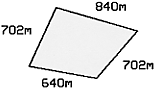a) 28.84 km
b) 2.884 km
c) 288.4 km
d) 2884 km

## Question 4

Find the distance around a circular flower bed that has radius of 4 feet.

a) 16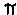ft
b) 8sq.ft
c) 9ft
d) 8ft

## Question 5

What is the area of a circular region whose diameter is 14 centimeters?

a) 28sq.cm
b) 14sq.cm
c) 196sq.cm
d) 49sq.cm

## Question 6

Find the surface area of a rectangular solid that is 15 inches long, 14 inches wide, and 5 inches high.

a) 1050 sq. in.
b) 710 sq. in.
c) 710 in.
d) 610 sq. in.

## Question 7

Find the volume in cubic centiliters of a 2.1-liter bottle.

a) 210 cubic cl
b) 2.1 cubic cl
c) 2100 cubic cl
d) 21 cubic cl

## Question 8

Find the volume of a rectangular solid that is 9 inches long, 8 inches wide, and 6 inches high.

a) 432 sq. in.
b) 486 cu. in.
c) 432 cu. in.
d) 348 in.

## Question 9

What type of measure is needed to express the area of the given circle?a) surface
b) square
c) linear
d) cubic

## Question 10

What type of measure is needed to express the perimeter of the triangle shown below?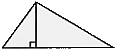a) cubic
b) surface
c) linear
d) square

## Question 11

What type of measure is needed to express the volume of the solid rectangle shown below?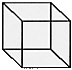a) cubic
b) linear
c) equilateral
d) square

## Question 12

Find the cost of carpeting an office that measures 21 feet by 24 feet if the carpet costs \$14.50 per square yard.

a) \$ 2436.00
b) \$ 7308.00
c) \$ 1218.00
d) \$ 812.00

## Question 13

The outside dimensions of a picture frame are 4 feet by 26 inches. If its inside dimensions are 2 2/3 feet by 25 inches, find the area of the frame.

a) 460 in.2
b) 448 in.2
c) 141.33 ft2
d) 45.67 ft2

## Question 14

A radio station is going to construct a 5-foot tower for a new antenna. The tower will be supported by three cables, each attached to the top of the tower and to points on the roof of the building that are 12 feet from the base of the tower. Find the total length of the three cables.

a) 52 ft
b) 65 ft
c) 13 ft
d) 39 ft

## Question 15

The city commission wants to construct a new street that connects Main Street and North Boulevard as shown in the diagram below. The construction cost has been estimated at \$120 per linear foot. Find the estimated cost for constructing the street.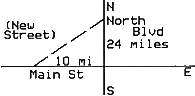a) \$ 81,120
b) \$ 633,600
c) \$ 16,473,600
d) \$ 137,280

## Question 16

For each of the figures below, S represents the sum of the measures of the interior angles. Study the given information, then find S for an eight-sided polygon.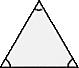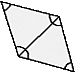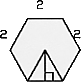3 sides 4 sides 6 sides 1 triangle 2 triangle 4 triangles S = 180 S = 360 S = 720

a) 1080
b) 900
c) 1440
d) 1260

## Question 17

Study the information given with the regular hexagons, then calculate the area of a regular hexagon with a side equal to 8.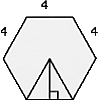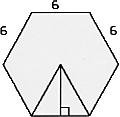area = 6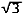area = 24area = 54a) 36b) 48c) 72d) 96## Question 18

Study the regular hexagon, then select the formula for computing the total area of the hexagon.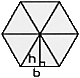a) A = 3h + b
b) A = 6hb
c) A = 3hb
d) A = 6(h + b)

## Question 19

Study the figure, then select the formula for computing the total area of the figure.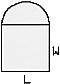a) A = 1/8L2 + LW
b) A = LW +L2
c) A =1/4L2 + LW
d) A =LW +W2

## Question 20

Study the trapezoid, then select the formula for computing the total area of the trapezoid.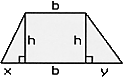B = b + x + y

a) A = 1/2 h(B + b)
b) A = b(B - x - y) + xh2
c) A = b(B - x - y)h
d) A = h(B + b)

## Question 21

Identify the angle below.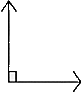a) straight
b) acute
c) right
d) obtuse

## Question 22

Select the geometric figure that possesses all of the following characteristics:

i.   polygon
ii.  three sides
iii. no sides with equal measure

a) scalene triangle
b) right triangle
c) equilateral
d) isosceles triangle

## Question 23

Which statement is true for the figure shown? (The notation "m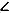A" indicates the measure of angle A.)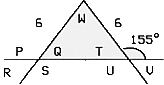a) mT = 25º
b) mW = mV
c) mW = 25º
d) mQ = 155º

## Question 24

Which statement is true for the figure shown, given that L1 and L2 are parallel lines?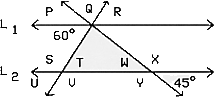```a) Since mT = 60º
mS = 115ºb) Since mT = 60º
mQ = 75º ```
c) None of the statements is true.
d) mV = mR

## Question 25

Study figures A, B, C, D. Then select the figure in which all triangles are similar.

a)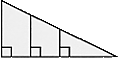b)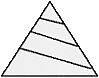c)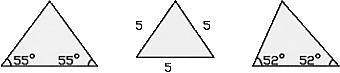d)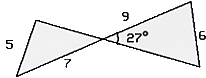## Question 26

Which statement is true for the pictured triangles?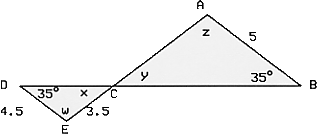a) CE/CA = CB/CD
b) mz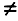mw
c) mx = 35º
d) AC = 3.9

## Question 27

Which statement is true for the pictured triangles?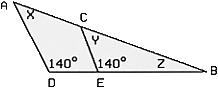a) None of these statements
b) mX = mZ
d) mX = mY

When you have completed this quiz, press the ALL DONE-CHECK TEST button below to check the test! Then you may go back and change the incorrect answers before you submit the test. You may check the answers as many times as you need until you achieve a perfect grade. Sometimes the pop-up box does not appear when you click on the button after checking the test the first time. If this happens, minimize the test and the answer box will be behind the browser. close the box and maximize the test to continue checking the test and finally submitting it.

When you have finished checking the test, press the SUBMIT button to send answers to your instructor